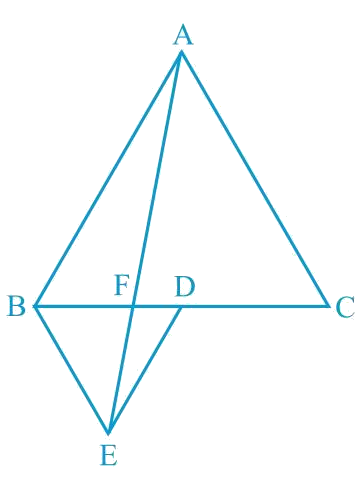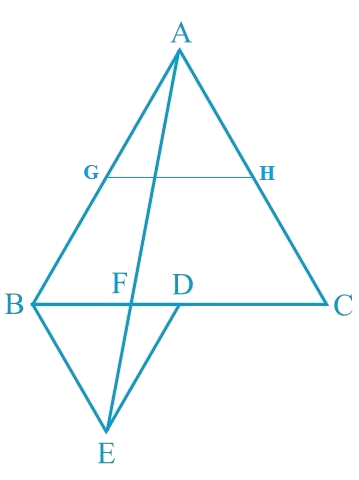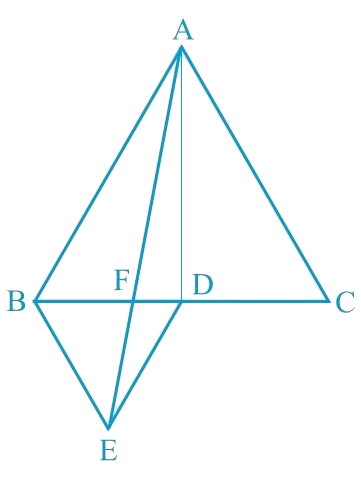# Ex.9.4 Q5 Areas of Parallelograms and Triangles Solution - NCERT Maths Class 9

Go back to  'Ex.9.4'

## Question

In the following figure, $$ABC$$ and $$BDE$$ are two equilateral triangles such that $$D$$ is the mid-point of $$BC$$. If $$AE$$ intersects $$BC$$ at $$F$$, show that

(i) \begin{align} \operatorname{ar}({BDE})=\frac{1}{4} \operatorname{ar}({ABC})\end{align}

(ii) \begin{align} \operatorname{ar}({BDE})=\frac{1}{2} \operatorname{ar}({BAE})\end{align}

(iii) $$\operatorname{ar}({ABC})=2 \text { ar }({BEC})$$

(iv) $$\operatorname{ar}({BFE})= \text { ar }({AFD})$$

(v) $$\operatorname{ar}({BFE})= 2 \text { ar }({FED})$$

(vi) \begin{align} \operatorname{ar}({FED})= \frac{1}{8} \text { ar }({AFC})\end{align}

Video Solution
Areas Of Parallelograms And Triangles
Ex 9.4 | Question 5

## Text Solution

What is known?

$$ABC$$ and $$BDE$$ are two equilateral triangles such that $$D$$ is the mid-point of $$BC. AE$$ intersects $$BC$$ at $$F$$.

What is unknown?

How we can show that

(i) \begin{align} \operatorname{ar}({BDE})=\frac{1}{4} \operatorname{ar}({ABC})\end{align}

(ii) \begin{align} \operatorname{ar}({BDE})=\frac{1}{2} \operatorname{ar}({BAE})\end{align}

(iii) $$\operatorname{ar}({ABC})=2 \text { ar }({BEC})$$

(iv) $$\operatorname{ar}({BFE})= \text { ar }({AFD})$$

(v) $$\operatorname{ar}({BFE})= 2 \text { ar }({FED})$$

(vi) \begin{align} \operatorname{ar}({FED})= \frac{1}{8} \text { ar }({AFC})\end{align}

Reasoning:

For the first part let $$G$$ and $$H$$ be the mid-points of side $$AB$$ and $$AC$$ and now we can use mid-point theorem. For remaining parts join vertices $$E$$ to $$C$$ and $$A$$ to $$D$$. Now we use the facts that median divides a triangle area in to two triangles of equal areas, If two triangles are on same base and between same pair of parallel lines then areas will be equal of both the triangles.

##[Hint: Join $$EC$$ and $$AD$$. Show that $$BE$$ $$\|$$ $$AC$$ and $$DE$$ $$\|$$ $$AB$$ etc.]

#### Steps:

(i) Let $$G$$ and $$H$$ be the mid-points of side $$AB$$ and $$AC$$ respectively.

Line segment $$GH$$ is joining the mid-points and is parallel to third side. Therefore, $$GH$$ will be half of the length of $$BC$$ (mid-point theorem).\begin{align}&\therefore {GH}=\frac{1}{2} BC \text{ and} \, {GH} \| {BD} \\ &\therefore {GH}={BD}={DC} \text { and } \\ \\ &{GH} \| {BD}({D} \text { is the mid-point of } {BC})\end{align}

Similarly,

• $$GD = HC = HA$$
• $$HD = AG = BG$$

Therefore, clearly $$\Delta$$$$ABC$$ is divided into $$4$$ equal equilateral triangles viz $$\Delta$$$$BGD$$, $$\Delta$$$$AGH$$, $$\Delta$$$$DHC$$ and $$\Delta$$ $$GHD$$

In other words,

\begin{align}\text { Area }(\Delta {BGD})=\frac{1}{4} \text { Area }(\Delta {ABC}) \end{align}

Now consider $$\Delta {BDG} \text { and } \Delta {BDE}$$

$$BD = BD$$ (Common base)

As both triangles are equilateral triangles, we can say

$$BG = BE$$

$$DG = DE$$

Therefore,

$$\Delta {BDG} \cong \Delta {BDE}$$ [By SSS congruency]

The SSS rule states that: If three sides of one triangle are equal to three sides of another triangle, then the triangles are congruent.

Thus, $$\text { area }(\Delta {BDG})=\text { area }(\Delta {BDE})$$

\begin{align} \therefore \operatorname{ar}(\Delta {BDE})=\frac{1}{4} \operatorname{ar}(\Delta {ABC}) \end{align}

Hence proved

##(ii) Area $$(\Delta {BDE})=$$ Area $$(\triangle {AED})$$  (Common base $$DE$$ and $$DE \| AB$$)

Area $$(\Delta {BDE})\, -$$ Area $$(\Delta {FED})\,=$$ Area $$(\Delta {AED})\, -$$ Area $$(\Delta {FED})$$

Area $$(\Delta {BEF})=$$ Area $$(\Delta {AFD}) \ldots (1)$$

Now,

Area $$(\Delta {ABD})=$$Area $$(\triangle {ABF})+$$ Area $$(\triangle {AFD})$$

Area $$( \Delta ABD ) =$$ Area $$( \Delta ABF) +$$ Area $$( \Delta BEF )$$ [ From equation ($$1$$) ]

Area $$(\Delta {ABD})=$$ Area $$(\Delta {ABE})$$  $$\ldots (2)$$

$$AD$$ is the median in $$\Delta {ABC}$$.

\begin{align} \text{ar}(\Delta {ABD}) &=\frac{1}{2} \text{ar}(\Delta {ABC}) \\ &=\frac{4}{2} \text{ar}(\Delta {BDE}) \\ & \quad \begin{pmatrix} \text{As }\\ \text{proved} \\ \text{ earlier } \end{pmatrix} \\ \text{ar}(\Delta {ABD})&=2 \text{ar}(\Delta {BDE}) \cdots (3) \end{align}

From Equation (2) and (3), we obtain

\begin{align} 2 \operatorname{ar}(\Delta {BDE})=\operatorname{ar}(\Delta {ABE}) \\ \operatorname{ar}({BDE})=\frac{1}{2} \text { ar }({BAE})\end{align}

(iii)

ar $$(\Delta {ABE})=$$ ar $$(\Delta {BEC})$$ (Common base $$BE$$ and $$BE \| AC$$ )

ar $$(\Delta ABF) +$$ar $$(\Delta BEF) =$$ ar $$(\Delta BEC)$$

Using equation $$(1)$$ we obtain

\begin{align}& \!\!\begin{pmatrix} \text{ar}(\Delta {ABF})\\+\operatorname{ar}(\Delta {AFD}) \end{pmatrix} \!\!=\operatorname{ar}(\Delta {BEC}) \\ &\operatorname{ar}(\Delta {ABD})=\operatorname{ar}(\Delta {BEC}) \\ &\frac{1}{2} \operatorname{ar}(\Delta {ABC})=\operatorname{ar}(\Delta {BEC}) \\ &\operatorname{ar}(\Delta {ABC})=2 \operatorname{ar}(\Delta {BEC})\end{align}

(iv) It is seen that $$\Delta BDE \text { and ar } \Delta {AED}$$ lie on the same base (DE) and between the parallels $$DE$$ and $$AB$$.

\begin{align} &\therefore \operatorname{ar}(\Delta {BDE})=\operatorname{ar}(\Delta {AED}) \\ \\&\therefore \operatorname{ar}(\Delta {BDE})-\operatorname{ar}(\Delta {FED}) \\ & \quad=\operatorname{ar}(\Delta {AED})-\text{ar}(\Delta {FED}) \end{align}

Subtracting ar $$(\Delta {FED})$$ on both the sides

$\therefore \operatorname{ar}(\Delta {BFE})=\operatorname{ar}(\Delta {AFD})$

(v) Let h be the height of vertex $$E$$, corresponding to the side $$BD$$ in $$\Delta BDE$$. Let $$H$$ be the height of vertex $$A$$, corresponding to the side $$BC$$ in $$\Delta ABC$$.

In (i), it was shown that

\begin{align} \operatorname{ar}({BDE})=\frac{1}{4} \operatorname{ar}({ABC})\end{align}

In (iv), it was shown that

ar $$(\Delta {BFE})=\operatorname{ar}(\Delta {AFD})$$.

$\therefore {\rm{ ar (}}\Delta BFE) = \text{ar} (\Delta AFD)$

${\rm{ = 2 ar (}}\Delta FED)$

Hence,

(vi)

\begin{align} & {\rm{ar }}\left( {\Delta AFC} \right) \\ &= {\rm{ar }}\left( {\Delta AFD} \right){\rm{ + ar }}\left( {\Delta ADC} \right) \\ &= \begin{pmatrix} 2 {\rm{ar }}\left( {\Delta FED} \right) \\ + \frac{1}{2}{\rm{ ar }}\left( {\Delta ABC} \right) \\ {\rm{ [using }}\left( {\rm{v}} \right)] \end{pmatrix}\end{align}

\begin{align} &= \begin{pmatrix} 2 \text{ar} \left( {\Delta FED} \right) \\+ \frac{1}{2} \left[ 4 \times {\rm{ar }}\left( {\Delta BDE} \right) \right] \end{pmatrix} \\ & \quad \left[ {{\text{Using result of part }}\left( {\rm{i}} \right)} \right] \end{align}

\begin{align} &= 2 \text{ar} \left( {\Delta FED} \right){\rm{ + 2 ar }}\left( {\Delta BDE} \right) \\ &= 2 \text{ar} \left( {\Delta FED} \right){\rm{ + 2 ar }}\left( {\Delta AED} \right)\\&= \begin{bmatrix} \Delta BDE \;\text{and} \;\Delta AED \\ \text{are on the same base} \\ \text{ and between same} \\ \text{ parallels}\end{bmatrix} \end{align}

\begin{align} &= \begin{pmatrix} 2 \text{ar}\left( \Delta FED \right) \\+ 2 \begin{bmatrix} {\rm{ar }}\left( \Delta AFD \right) \\ + {\rm{ ar }}\left( \Delta FED \right) \end{bmatrix} \end{pmatrix} \\ &= \begin{pmatrix}2 \text{ar} \left( {\Delta FED} \right) \\+ 2 {\rm{ ar }}\left( {\Delta AFD} \right) \\+ 2 {\rm{ ar }}\left( {\Delta FED} \right) \\ \left[ {{\rm{Using }}\left( {{\rm{viii}}} \right)} \right] \\ \end{pmatrix} \\ &= 4 \text{ar} \left( {\Delta {{FED}}} \right){{ + 4 ar }}\left( {\Delta {{FED}}} \right)\\&\Rightarrow {\rm{ar }}\left( {\Delta {{AFC}}} \right)\rm = 8 ar \left( {\Delta {{FED}}} \right)\\&\Rightarrow {\rm{ar }}\left( {\Delta {{FED}}} \right){\rm = }\frac{1}{8}{\rm{ ar }}\left( {\Delta {{AFC}}} \right) \end{align}

Learn from the best math teachers and top your exams

• Live one on one classroom and doubt clearing
• Practice worksheets in and after class for conceptual clarity
• Personalized curriculum to keep up with school